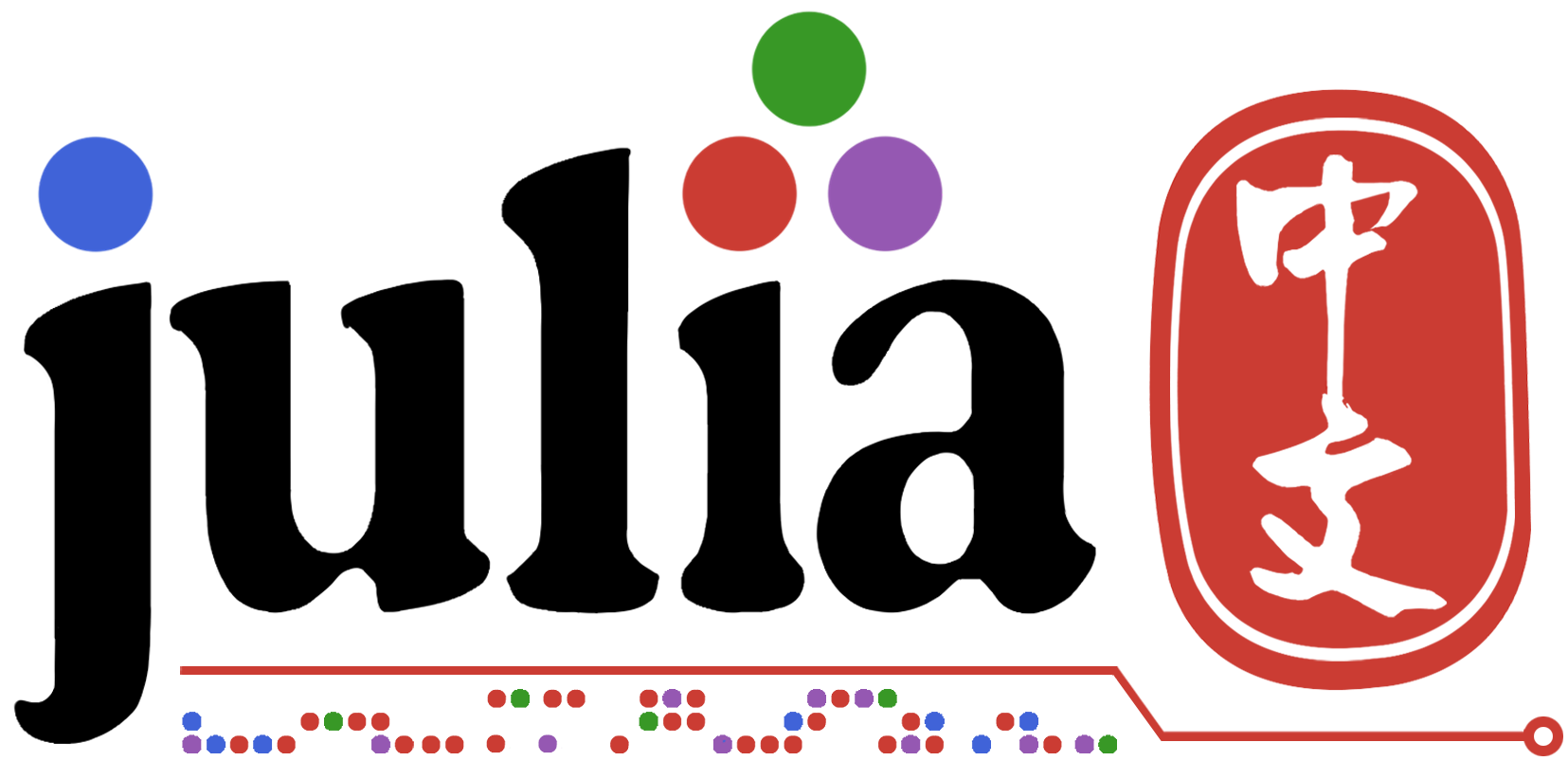# 标准差函数std的使用

std(itr; corrected::Bool=true, mean=nothing[, dims])

Compute the sample standard deviation of collection itr.
The algorithm returns an estimator of the generative distribution’s standard deviation under the assumption that each entry of itr is an IID drawn from that generative distribution. For arrays, this computation is equivalent to calculating sqrt(sum((itr .- mean(itr)).^2) / (length(itr) - 1)). If corrected is true, then the sum is scaled with n-1, whereas the sum is scaled with n if corrected is false with n the number of elements in itr.

std(A,true), std(A;true)都不对？

`;` 分隔了位置参数和关键字参数，关键字参数需要给出具体名称

``````std(A, corrected = true)

# Julia 1.5 以后变量名可以作为关键字参数直接传入
corrected = true
std(a; corrected) # 等价于 std(a; corrected=corrected)
``````
2赞